Keyah Math Module 12, Level 4

Mathematical Content: Exponential and logarithmic functions, algebraic operations, graphs

#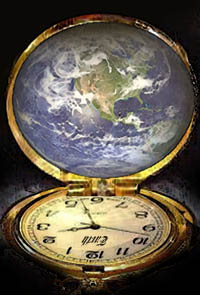Finding the Age of the Earth: Level 4

Introduction
How can we tell how old the Earth is?
Certain natural phenomena or processes, such as Earth's year-long solar orbit, and the resulting annual climatic variations that govern the growth of tree rings, can be used as "natural clocks."
If we can find and date a rock that we know has been around since the Earth formed, we can measure the age of the Earth. Can we find in rocks a natural clock that has been operating since they formed? It was discovered that some chemical elements, notably uranium and thorium, are strongly radioactive. These elements occur naturally in nearly all rocks, and they account for the radioactivity you could observe with a Geiger counter.

The radioactive decay process can be described simply as the transformation of an unstable radioactive atom (called the parent) to a new atom (called the daughter) that may differ in atomic number, atomic mass, or both. The transformation occurs either by loss of particles from, or addition of particles to, the parent nucleus.

In some parent-daughter pairs, the daughter is still radioactive and subject to further decay to a new daughter. In other cases, decay yields a daughter that is non-radioactive (stable) and will remain unchanged for the rest of time. The time interval it takes for the parent atoms to decay by half is always the same, no matter how much of the parent element remains. This constant length of time is called the half-life.

How does radioactive decay serve as a "natural clock"?
Some common rocks are weakly radioactive. Numerous chemical analyses of crustal rocks have revealed that radioactive isotopes of elements such as uranium, thorium, potassium, and rubidium occur naturally in these rocks and account for their radioactivity. The precise half-lives of these isotopes have been measured experimentally.

These radioactive isotopes and their half-lives can be used as our natural clock, i.e., we can find out how old certain rocks are from this information.

Part 1.        Using Math to Find the Age of Rock in Southwest Colorado

In this section, we will guide you through the process of finding the decay constant for a radioactive isotope in the basic decay equation, and then use this and another decay equation to find the age of a sample of gabbro found at Electra Lake, just north of Durango in southwest Colorado. Following this, you can repeat these steps to estimate the age of the Earth.

Rubidium-Strontium Dating
Rubidium (87Rb) decays to strontium (87Sr) and because the half-life is so long, it is used by geologists to find the age of very old rock. The isotopes that are used for dating are 87Rb, 87Sr, and 86Sr.  87Rb decays to 87Sr; 86Sr is not a product of decay but is used as a reference isotope. This isotope system can be used as a natural clock to determine the age of many old rocks. This method is called Rubidium-Strontium dating by geologists.

The decay function is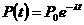, where

P(t) = number of atoms of the parent isotope at time t,
P0 = P(0) = initial number of atoms of the parent isotope  (at t = 0, when decay started),
λ = (Greek letter lambda) the decay constant specific to that parent isotope, which can be determined experimentally, and is equivalent to the fraction of atoms that decays per some interval of time.

We can use the basic decay equation and isotope half-life to find the decay constant for specific isotopes.

Finding the decay constant λ
Below is a table of the parent-daughter pair (or isotope system) that we will use in radiometric dating of the Electra Lake gabbro and the Earth.  The half-life is given in million (106) years.

 Isotope System Half-life T Parent isotope (symbol) Daughter isotope (symbol) (in million years) Rubidium-87 (87 Rb) Strontium-87 (87 Sr) 4.88 x 104
 Question 1.   What is the value of the decay constant for rubidium-strontium?

For a worked solution to this question, click here.

The decay constant λ = 1.42 x10-5 for the Rb-Sr system.

 Question 2.   Suppose that in an isotope system, the parent decays into the daughter, and there was no daughter atoms present initially. What is the ratio of daughter atoms to parent atoms at time t?

For a worked solution to this question, click here.
This solution to Question 2,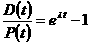, shows the desired ratio—note that this is true for any such isotope system--this equation is called the basic decay equation.

Modification of the Basic Decay Equation for Rb-Sr Dating
First, solve the basic decay equation for the number of daughter atoms, D(t).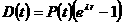Rocks that contain 87Rb also contain initial amounts of 87Sr, so when comparing the relative amounts of 87Rb and 87Sr, the amount of 87Sr present initially must be accounted for. Also, a certain amount of 86Sr is present that is not a product of Rb decay, this is stable.
So when counting the amount of the daughter 87Sr, the basic decay equation must be modified to add in the amount present initially, 87Srinitial, to the amount decayed at time t, 87Srt. This gives the modified equation,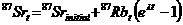.
At time t = now, the ratio of both 87Srt and 87Rbt to 86Sr can be measured, so divide both sides of the above equation by 86Sr (which is constant) to get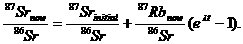This equation has the form of a linear equation y = b + mx, where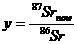,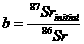,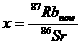, and slope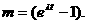Now, Rb-Sr dating of a rock incorporates the following procedure that is followed below:

• Rock samples are collected from a site

• The ratios x and y are measured in each sample with a mass spectrometer

• The results are plotted on an (x, y)-axis system (this plot is called an isochron diagram)

• The line of best fit for the plot is found using linear regression

• The slope of this line is set equal to m =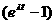• The value of t is found from this equation—this is the age of the rock!

(Reference: Looking into the Earth; Musset & Khan; Cambridge University Press 2000)
Information: Five samples of gabbro were colleted near Electra Lake in southwest Colorado. Ratios x and y were measured by a mass spectrometer and are listed in the table below.

(Reference: Precambrian Rb-Sr Chronology in the Needle Mountains, Southwestern Colorado; Bickford, Wetherill, Barker, Lee-Hu; J. Geophysical Research, Vol. 74, No. 6, 1969)

 X Y 1.731 0.7415 0.580 0.7155 0.135 0.7078 0.569 0.7172 3.198 0.7700

The graph shown below indicates the points plotted, the regression line and its equation. (This graph is from the linear regression applet.)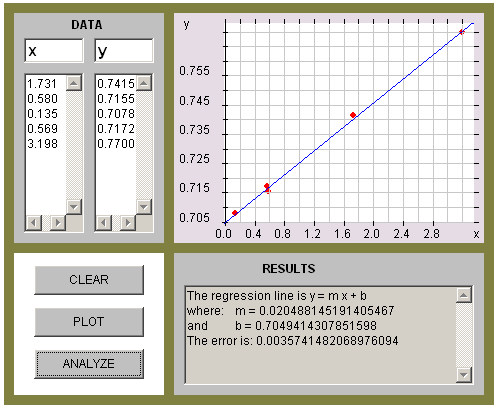The equation of the regression line is
Y = 0.7049 + 0.0205x,
its slope is m = 0.0205.

 Question 3.   What is the age of the gabbro from Electra Lake?

Answer to Question 3. The gabbro is, approximately, 1,429 million years old

Part 2.        Using Math to Find the Age of the Earth
Here you will use Rubidium-Strontium decay to date a meteorite samples. Assuming that samples, the Earth, and the entire solar system were formed at approximately the same time, this should give us a good approximation to the age of the Earth.

From five meteorite samples, a mass spectrometer measures the ratios of 87Srnow to 86Sr and 87Rbnow to 86Sr; the data are listed in the table below.

 Meteorites 87Rb/86Sr 87Sr/86Sr Modoc 0.86 0.757 Homestead 0.80 0.751 Bruderheim 0.72 0.747 Kyushu 0.60 0.739 Buth Furnace 0.09 0.706

(Reference: Rubidium-Strontium Dating of Meteorites; http://hyperphysics.phy-astr.gsu.edu/hbase/nuclear/meteorrbsr.html)

 Question.   How old is the Earth?

In order to answer this question, you can repeat the procedure given in the dating of the gabbro; specifically:

• Plot the data on an (x, y)-axis system to create the isochron diagram
• Use linear regression to find the slope of the line of best fit
• Set  the slope equal to• Solve for t.

(You can solve this either algebraically or graphically—ask your Instructor. You may be provided a print-out of a graph that can be used to find the answer to this question, or you can use a graphing calculator, or go to the Kéyah Math or Earth Math website and use the Plot/Solve applet. )

This material is based upon work supported by the National Science Foundation under Grant GEO-0355224. Any opinions, findings, and conclusions or recommendations expressed in this material are those of the authors and do not necessarily reflect the views of the National Science Foundation.